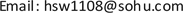1. 引言

Terzaghi (1923)根据实验室测量(图1)，提出含水饱和土壤的有效应力定理，土壤在应力条件下的应变和破坏取决于压力差(有效应力) 

P p = P c − P d (1)

Biot (1941)建立了饱含水固结黏土的三维力学模型，模型中的参数比较抽象，不是很明确  。后来Biot和Willis (1957)基于低渗透性多孔介质(例如岩石)提出了修正后的有效应力定理，由此引入有效应力系数的定义  ，即

n P p = P c − P e n = 1 − K d r y K m a } (2)

Zimmerman (1991)从二维力平衡的角度推导出岩石基质的平均应力(有效应力)表达式(图2) 

P c = ( 1 − ϕ ) P ¯ + ϕ P p (3)

2. 围压P<sub>c</sub>

3. 有效应力P<sub>e</sub>

P e 1 = σ 1 − P p P e 2 = σ 2 − P p P e 3 = σ 3 − P p } (4)

P e 1 = σ 1 − n P p P e 2 = σ 2 − n P p P e 3 = σ 3 − n P p } (5)

P e 3 = P o v − n P p (6)

P e 3 = P o v − P p (7)

P e 3 = f ( V ) (8)

4. 有效应力系数n

Prediction method of pore pressure based on P-wave velocit

Eaton (1972) P p = P o v − ( P o v − P h ) ( V V n ) NVn为正常压力下的速度，V为实测地层页岩或泥岩的速度，Pp为预测的孔隙压力，Pov为上覆岩层压力，Ph为静水柱压力(正常压力)，N为经验系数，在墨西哥湾N值通常为3.0。
Traugott(1994) P p = ( P o v − P h ) ( 1 − ϕ v 1 − ϕ n ) xx是经验系数，fv是由页岩声波时差确定的孔隙度，fn是由正常压实趋势确定的孔隙度，Ph为静水压力(正常压力)。
Flemings和Stum等(2002) P p = P o v − [ 1 k ln ( ϕ 0 ϕ ) ] ϕ = 1 − ( Δ t m a Δ t ) 1 / fPp为预测的地层孔隙压力，Pov为上覆地层压力，f0是初始孔隙度(有效应力为零)，k是与地层压实有关的常数，f是声波地层因子，f是地层孔隙度， Δtma是岩石基质的声波时差。

Phillppone (1979) P p = V max − V int V max − V min P o vPp为预测的地层孔隙压力，Pov为上覆地层压力，Vmax接近于岩石刚性基质的速度(岩石骨架速度)，Vmin接近于岩石孔隙流体速度，Vint为地层层速度。c为与之对应层速度有关的系数 c = a e b V int ，a、b为常数。
Phillppone (1982)修正式 P p = c ( V max − V int ) ( V max − V min ) P o v
Bowers (1995) 加载V = V 0 + A σ B , Math_35#V是岩石速度，V0为有效应力为零时的速度，σ为有效应力，Pp为预测的地层孔隙压力，Pov为上覆地层压力，A和B为经验常数，不同的地区V0、A和B一般不一样。σmax和Vmax是卸载初始的有效应力和速度，U是反映沉积岩的弹性参数。

Wang等人(2016)  根据Pickett (1963)和他人的实验结果  ，干燥岩石的泊松比常常近似地等于矿物的泊松比，或Kdry/Kma = μdryma (Mavko et al. 1998, p. 244)  的关系联立Biot-Gassmann 原理(Gassmann, 1951  ; Biot, 1956  )推导出一种新的估算有效应力系数的方法。

a K m a 2 + b K m a + c = 0 a = ϕ K f ( K s a t + 4 3 μ s a t ) − 1 b = ρ m a V m a 2 ( 1 − ϕ K s a t K f ) + ( 1 − ϕ ) K s a t − 4 3 ( 1 + ϕ ) μ s a t c = K s a t ( 4 3 μ s a t − ρ m a V m a 2 + ϕ ρ m a V m a 2 ) K m a = − b + b 2 − 4 a c 2 a K d r y K m a = K s a t K m a [ 1 + ϕ ( K m a K f − 1 ) ] − 1 K s a t K m a + [ 1 + ϕ ( K m a K f − 1 ) ] − 2 } (9)

Norris (1989)给出了有效应力系数的一般边界为 

3 ϕ 2 + ϕ ≤ n ≤ 1 (10)

5. 孔隙流体压力P<sub>p</sub>

Comparison of genesis of abnormal high pressure both at home and abroa

Swarbrick Osborne (1998) 应力相关型

Swarbrick sborne和Yardley (2002) 应力相关型：垂向应力型、侧向应力型

Chilingar Serebryakov和Robertson等(2002) 岩石孔隙体积的变化(如欠压实)

(砂岩)的孔隙流体压力。然而在很多情况下，砂岩孔隙中的压力与页岩夹层的压力没有直接的关系，假如存在直接的关系，可能会导致严重的钻井事故。因为超压页岩中的砂岩目的层压力可能比周围页岩低，稍有不慎，就有可能由于过重的补偿泥浆将砂岩压漏。预测的地层压力和测量的地层压力之间的关系是非常复杂的，涉及到地质背景的分析和烃类的预测  。而Shaker (2002)  指出了目前地层压力预测最根本的问题，如一些基于泥岩或页岩欠压实理论的方法，预测的是泥岩或页岩的孔隙流体压力(非渗透性地层压力)，而实际测量的是储层类岩石(如砂岩)的孔隙压力(渗透性地层压力) (王鸿升，2016)  。这就涉及到岩石的孔隙是否连通的问题，如果岩石的渗透率接近于零，岩石中的各孔隙互相不连通(图5)，那

Biot和Willis (1957)  提出的修正后的有效应力定理式(2)是否适用于非渗透性地层的孔隙流体压力计算，到目前没有一个肯定的结论。在一般情况下，泥岩和页岩的渗透率很低，可接近于零。Mitchel指出结合水的结构不同于一般水，但结合水在水动力特性与一般水没有本质的区别，达西定律还是适用的  。李广信(2011)通过实验室测量发现，黏性土结合水是能传递水压力的,但是其力学机制难以从应变与应力的关系来进行解释  。

6. 结论